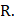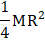# One quarter sector is cut from a uniform circular disc of radiusThis sector has mass. It is made to rotate about a line perpendicular to its plane and passing through the centre of the original disc. Its moment of inertia about the axis of rotation isa)b)c)d)## Question ID - 100625 :- One quarter sector is cut from a uniform circular disc of radiusThis sector has mass. It is made to rotate about a line perpendicular to its plane and passing through the centre of the original disc. Its moment of inertia about the axis of rotation isa)b)c)d)3537

(a)

We cannot consider the quadrant as a single mass as the distance of different particles is different from the axis of rotation. So we take the help of calculus. Let us consider a segment as shown in Figure. All masses lying in this segment are at a distancefrom the axis and hence considerd as a small differential mass. Let the thickness of the segment beThe mass per unit area of the quadrant =Area of the segmentMass of the segmentMI of this segment aboutMI of the quadrant aboutNext Question :

Complete the following number series by choosing the correct answer from the given alternatives.

 113, 114, 118, ?, 143, 168 (a) 127 (b) 129 (c) 134 (d) 139